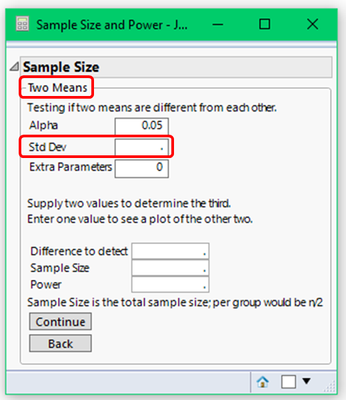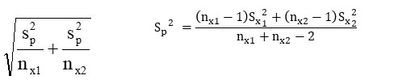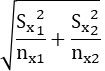Choose Language Hide Translation Bar
Highlighted

## Power & Sample Size For Testing Two Means : Input for StdevDear JMP Community,

I am trying to evaluate the Power & Sample Size for Testing Two Means.

Above is the platform I used to evaluate this.

My question:

How should I input for Stdev? I understood it should be from historical data.

However, because I have 2 group of data (hence Testing for Two Means), which stdev data should I input?

For example, the descriptive statistics for these 2 groups of data could be like:

Group 1 Data : Mean1 and Stdev1.

Group 2 Data : Mean2 and Stdev2.

Should I input Stdev1 value, or Stdev2 value?

or

Should we input stdev based on whether the Variance is equal or not?

If Equal Variance, the Stdev would be calculated as:If Unequal Variance, the Stdev would be calculated as:B.r,

Chris Looi

3 REPLIES 3
Highlighted

## Re: Power & Sample Size For Testing Two Means : Input for Stdev

You assume the variances are equal. The sample size calculations will provide a sample size that is then split equally between the two groups.
Dan Obermiller
Highlighted

## Re: Power & Sample Size For Testing Two Means : Input for Stdev

Hi Dan,

Do you mean the value of Stdev needed to be input (based on historical data) need to be computed first as below (since we have to assume variances are equal)?

The stdev would be, when variance are equal:B.r,

Chris Looi

Highlighted

## Re: Power & Sample Size For Testing Two Means : Input for Stdev

The platform assumes equal variance, so the value you would put in for Std Dev is Sp. For conservatism, you may choose to use Sp to get an upper confidence bound (90% or 95% perhaps) on Std Dev and use that as your assumed Std Dev.

Article Labels

There are no labels assigned to this post.